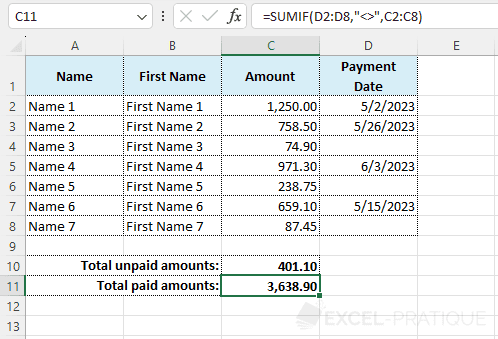# Excel Function: SUMIF

The Excel function SUMIF allows you to perform a sum taking into account a criteria.

Usage:

`=SUMIF(range, criteria)`

or (if the range for the sum is different from the criteria range)

`=SUMIF(range, criteria, sum_range)`

## Usage Example

The goal here is to calculate the total of unpaid amounts.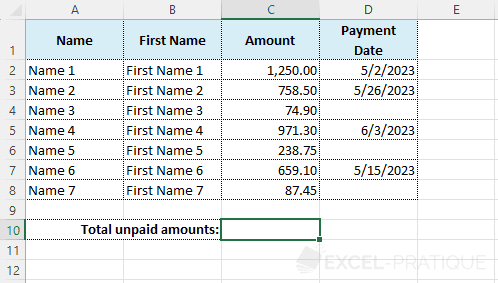To differentiate between paid and unpaid amounts, we will check if the payment date is filled in or not.

To do this, click on cell C10 and select the SUMIF function:

• Range: the data range in which the criteria will be checked (here, the payment dates)
• Criteria: the "" criteria (to indicate that the payment date should be blank)
• Sum_range: the data range that will be used for the sum calculation (here, the amounts)
``=SUMIF(D2:D8,"",C2:C8)``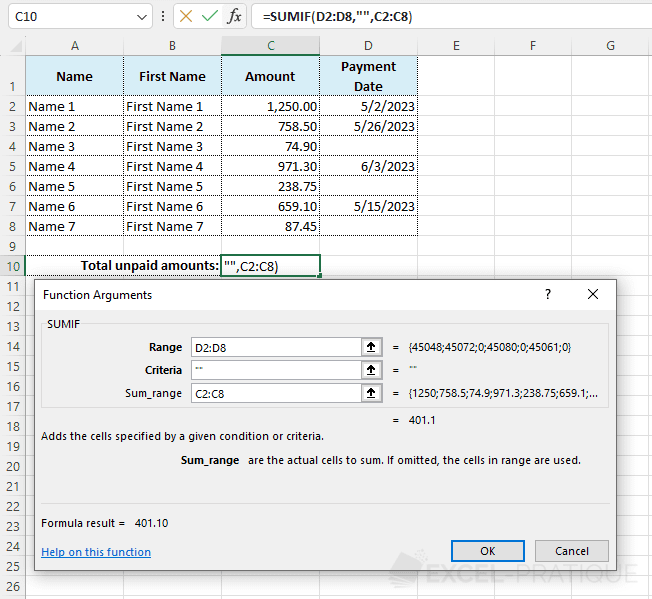Click OK.

In this case, Excel will sum all the amounts for which the payment date is blank.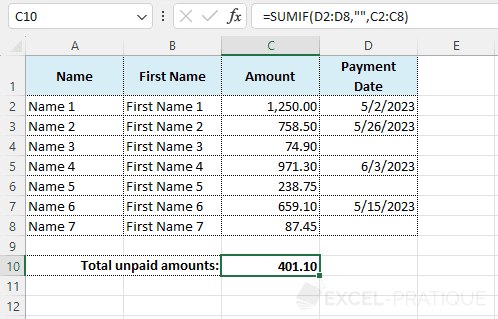To get the total of paid amounts, you can use the same formula replacing the "" (empty) criteria with the opposite "<>" (not empty) criteria:

``=SUMIF(D2:D8,"<>",C2:C8)``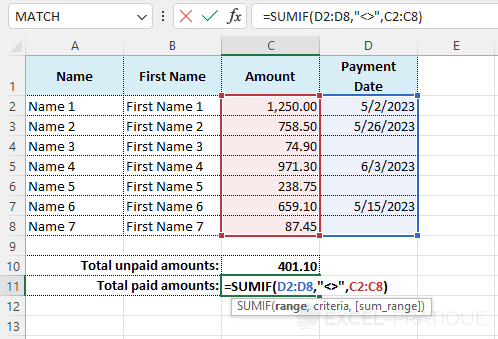To get: